Choose Language Hide Translation Bar
Variable clustering in JMP

When presented with a large number of variables to predict an outcome, you may want to reduce the number of variables in some way to make the prediction problem easier to tackle. One possible dimension reduction technique is the well-known method of principal components analysis (PCA). The variables resulting from PCA, however, can be hard to interpret. An alternative strategy is to use variable clustering, a method available in JMP Pro.

Created by SAS/STAT Development Director Warren Sarle for the VARCLUS procedure, variable clustering reduces the number of variables by grouping similar variables together. This lets you use the resulting cluster components, the first principal component from variables within each cluster, as new variables. These new variables share a similarity in that they are only made up of variables within each cluster. To further improve interpretation while reducing collinearity, you can alternatively use only the most representative variable from each cluster in place of each cluster component.

How Variable Clustering Works

The variable clustering algorithm is an iterative algorithm that begins with all variables in a single cluster, and it proceeds by iteratively splitting and assigning variables to new clusters until no new splits or assignments are possible. More specifically, clusters are iteratively split into smaller clusters according to the principal components of the clusters. At each iteration of the algorithm, the cluster with the largest second eigenvalue is chosen to be split into two new clusters. The members of the cluster being split are assigned to the new clusters based on their correlation to the first two orthoblique rotated principal components of the cluster being split. Once split, all other variables are examined and reassigned to a different cluster if it is found to have a higher correlation with a different cluster. Variable clustering ends once the second eigenvalue of all clusters is less than one.

Example: Predicting Wine Quality

To demonstrate variable clustering, let’s predict wine quality using the wine quality data set provided at the UCI Machine Learning Repository. Separated by red and white wines, this data set consists of the following 11 variables describing the contents of each wine: fixed acidity, volatile acidity, citric acid, residual sugar, chlorides, free sulfur dioxide, total sulfur dioxide, density, pH, sulphates and alcohol.

To reduce the dimension of these variables with variable clustering, we first launch the Principal Components platform from the Analyze menu. Next, we select the variables as Y, Columns and click OK.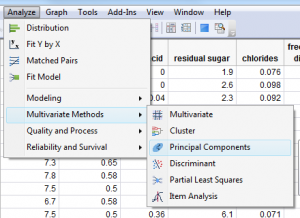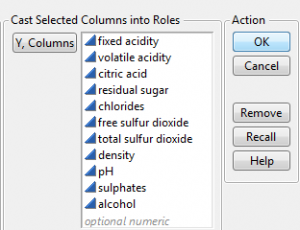This launches the Principal Components platform for the wine quality data. From here, select the Cluster Variables option under the Principal Components red triangle menu. You should see the Variable Clustering cluster summary. As this summary shows, the 11 variables have been summarized by four clusters, with each cluster having 4, 3, 2, and 2 members, respectively. The Most Representative Variable column shows the variable that best represents each cluster of variables. The proportion of variation explained within each cluster is listed in the column Proportion of Variation Explained whereas the overall proportion of variation explained, 0.558, is listed at the bottom of the summary.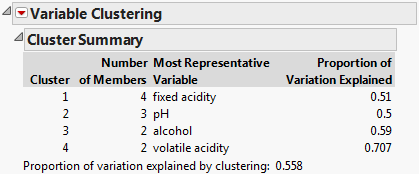We can request a table of cluster members using the Variable Clustering red triangle menu. These cluster members give insight into the similarity between variables. For example, this table shows that the four members of cluster number 1 are similar: fixed acidity, chlorides, density and sulphates.

The RSquare between each variable and the cluster it is a member of is listed in the column RSquare with Own Cluster. The RSquare with Next Closest column is the RSquare between each variable and the next most similar cluster. The 1-RSquare Ratio column is a measure of relative closeness between the cluster a variable is a member of and the next most similar cluster. For example, the 1-RSQuare Ratio for fixed acidity is computed with (1-0.527)/(1-0.106) = 0.473/0.894 = 0.529. This illustrates how this quantity measures relative closeness. A value of 1-RSquare Ratio greater than 1 would mean that the next closest cluster is actually more similar than the cluster the variable is currently a member of, and as a result all 1-RSquare Ratios should be less than 1.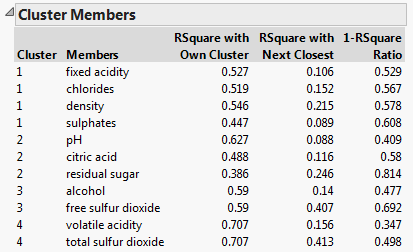Wine Quality Reduction Comparison

To compare how these various reduction techniques predict wine quality, we fit a linear regression using three different sets of predictor variables:

• The first four principal components (PCs).

• The four cluster components (CCs).

• The four most representative variables from each cluster (MRVs).

These models are fit to a two-thirds training data set, and we use the Model Comparison platform to compare the models.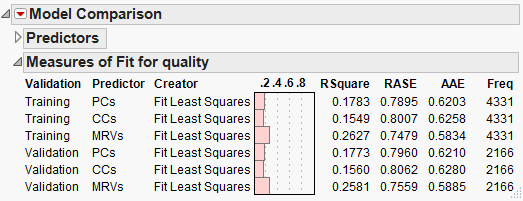The training sample RSQuare and validation sample RSquare tell a similar story: The model with principal components slightly outperforms the model with cluster components, while the model using the most representative variables outperforms both. This is an ideal solution, as we have not only found a more parsimonious model, but we have also found one that provides an easier interpretation of results.

Consider adding variable clustering from JMP Pro to your variable reduction toolkit today!

References

P. Cortez, A. Cerdeira, F. Almeida, T. Matos and J. Reis. Modeling wine preferences by data mining from physicochemical properties. In Decision Support Systems, Elsevier, 47(4):547-553, 2009.

Article Labels

There are no labels assigned to this post.

Article Tags
Visitor

Jose Guerrero wrote:

Hello,

Do we have an option to choose the number of clusters as we have in VARCLUS for JMP 11 pro PC

ThanksStaff

Ryan Parker wrote:

Hi Jose, this is not currently an option in JMP 11 Pro. We will plan to add this future in a future version of JMP Pro!

Ryan

Level I

The clustering of variables function has been really helpful for my work.

Can you tell me how the final number of clusters are controlled - which criteria are used to make this decision i.e. the eigenvalue?

For JMP 14 are you considering to include an option to choose the number of clusters?

Also, it would be nice to have a dendrogram or some kind of visual to show the relationship

Lene